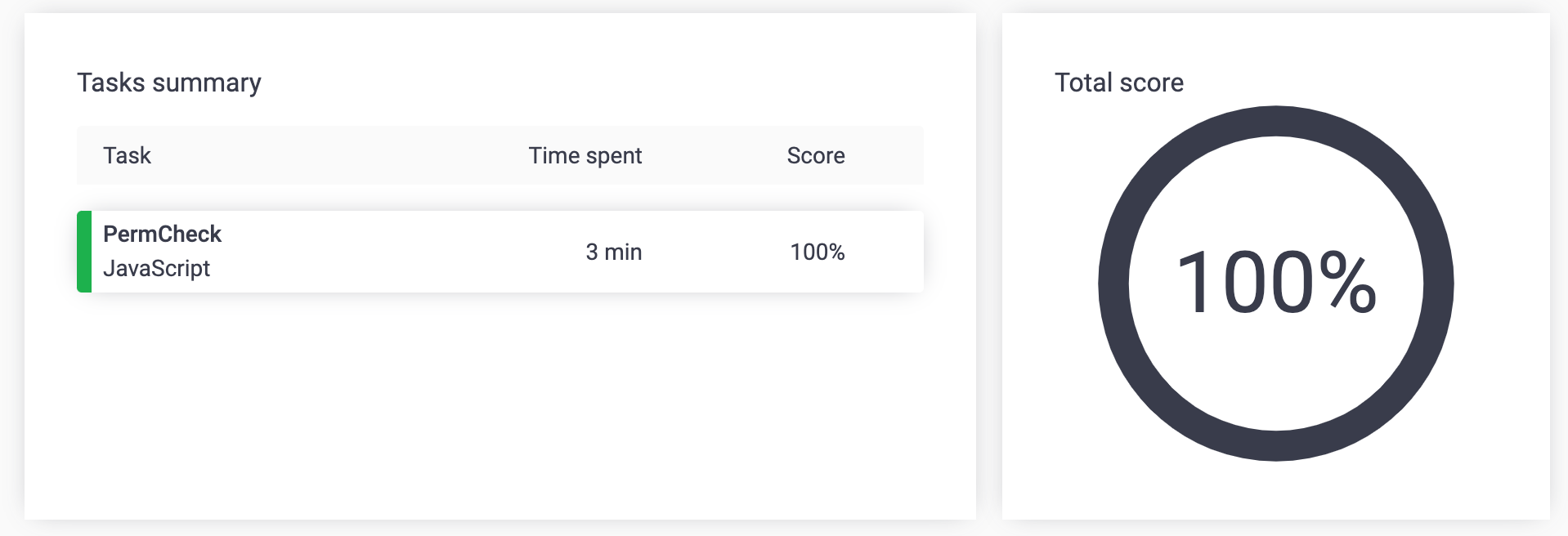# 알고리즘 PermCheck

taewo·2020년 6월 26일
0

A non-empty array A consisting of N integers is given.

A permutation is a sequence containing each element from 1 to N once, and only once.

For example, array A such that:

A = 4
A = 1
A = 3
A = 2

is a permutation, but array A such that:

A = 4
A = 1
A = 3

is not a permutation, because value 2 is missing.

The goal is to check whether array A is a permutation.

Write a function:

function solution(A);

that, given an array A, returns 1 if array A is a permutation and 0 if it is not.

For example, given array A such that:

A = 4
A = 1
A = 3
A = 2

the function should return 1.

Given array A such that:

A = 4
A = 1
A = 3

the function should return 0.

Write an efficient algorithm for the following assumptions:

N is an integer within the range [1..100,000];
each element of array A is an integer within the range [1..1,000,000,000].

function solution(A) {
// write your code in JavaScript (Node.js 8.9.4)
let pivot = 1
const sortA = A.sort((a,b) => a-b)
for (let i=0; i<A.length; i++) {
if (i+1 !== A[i]) {
pivot = 0
break
}
}
return pivot
}